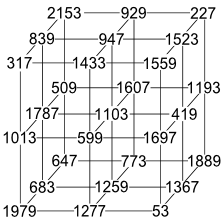## Friday, January 4, 2013

### 2153

2153 is a prime number.

2153 is the sum of five consecutive composite numbers (A060330): 2153 = 428 + 429 + 430 + 432 + 434.

2153 has a representation as a sum of two squares: 2153 = 282 + 372.

2153 is the hypotenuse of a primitive Pythagorean triple: 21532 = 5852 + 20722.

In 1977, Akio Suzuki used primes ranging from 53 to 2153 to construct the magic cube with the smallest possible magic sum (for cubes with side three and distinct prime entries).Source: Prime Curios!